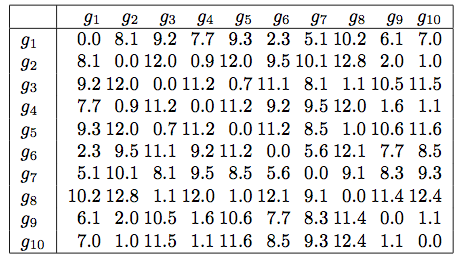# Glossary

## Distance matrix

A distance matrix is a matrix $D$ whose entries encode distances between pairs of taxa. Formally, $D_{j,k}$ represents the distance between the $j$th and $k$th taxa, where the choice of particular distance metric may vary. Distance matrices are widely used in distance-based phylogenetic methods.

Commonly used distances encoded in matrices include comparison of genetic strings by Hamming or edit distance, morphometric analysis, and various formulae (such as Euclidean distance) applied to comparing characters. For extension to the creation of a phylogeny, we demand that the underlying matrix be consistent.

The figure below shows an example of a distance matrix whose distances quantify the similarity between pairs of genes based on patterns of expression.